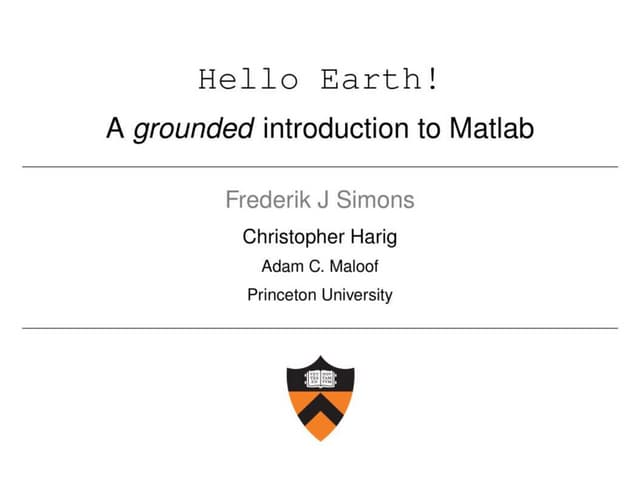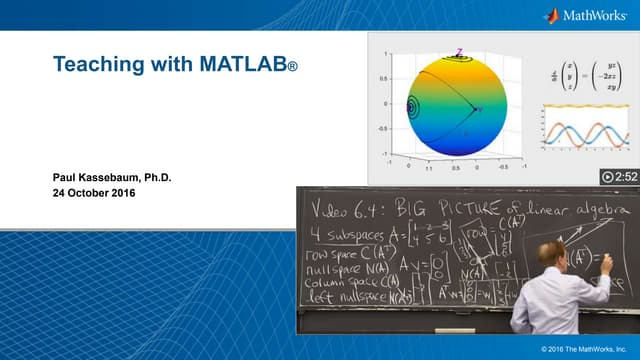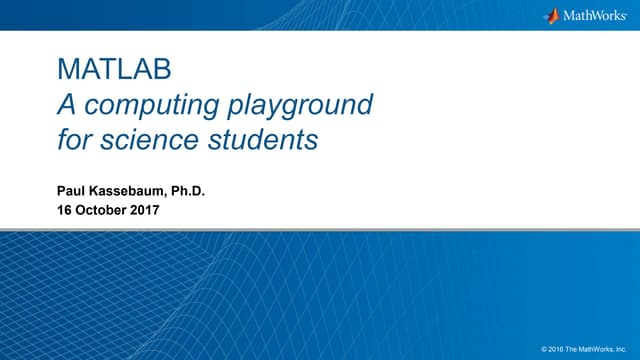# Computational Thinking

Content on this page was originally created for On the Cutting Edge: Teaching with Data, Simulations, and Models and is expanded here. Other content is derived from participant presentations, discussions, and breakout groups at the Teaching Computation with MATLAB workshops and benefitted from the editing of Risa Madoff, University of North Dakota.

Computational thinking is the thought processes associated with examining a problem and formulating a solution that can be solved by a person or machine. More and more, scientists are turning to machines and programs to tackle complex problems and large datasets, requiring strong computation and programming skills.

Students who learn computational thinking and the skills to take advantage of programs such as MATLAB can take control of their data, explore models, and output results with a complete understanding of what happened. While most students do not become programmers, it is important for them to understand how computation and modeling contribute to scientific problem solving. With a little understanding and groundwork, a non-programmer can know enough to ask probing questions about programming output. Educators can ensure the development of these skills by exposing students to computation and programming through focused, scaffolded, and contextualized assignments that allow them to explore problems and the tools scientists use to solve them.

Jump down to: Getting Started with MATLAB | Resources

## Introducing Students to ComputationClick to view
Frederik Simons (Princeton University) presented his approach to introducing students to MATLAB at the 2015 Workshop: Hello Earth! A grounded introduction to MATLAB. Simons focuses on building programming skills and uses many engaged pedagogies including teaching with data and incorporating research-like experiences. His methods launch students into utilizing many MATLAB features and thinking like an expert.

### Other approaches discussed at the workshop included:

• Producing simple plots
• Illustrating that the program produces things they are familiar with
• Exploring the anatomy of a program - what is it?
• Assigning variables/using command line
• Scripts, functions
• Diagramming - coding with intention
• Creating visual representations of finding a solution to a problem
• Metacognitive opportunities
• Reflection about a problem, such as calculations, correlations, spatial and temporal scales, dimensionality, conversion, functionality, and error
• Written diaries recording and reflecting on code, syntax, and programming

## Getting Started with MATLAB

During the 2016 and 2017 workshops on teaching computation with MATLAB, Paul Kassebaum (MathWorks) discussed the value of computational thinking and tools to get students started with MATLAB. He also discussed resources on the MathWorks website that can be useful for implementing computation using MATLAB in your courses.

### Paul Kassebaum's Presentations:Click to view
In his 2016 presentation, Teaching with MATLAB, Paul discussed ways to incorporate and improve the use MATLAB teaching. He covers course content, pedagogical methods, course structure, materials and implementation, and faculty development and support.Click to view
In his 2017 presentation, MATLAB: A computing playground for science students, Paul showed how MATLAB can be used as a "computing playground" where students are able to interactively build code, run simulations, solve problems numerically or analytically, extract, analyze, and visualize experimental data, model, and simulate phenomena to build intuition, express and simulate equations to test hypotheses, simulate analytical models to test predictions.